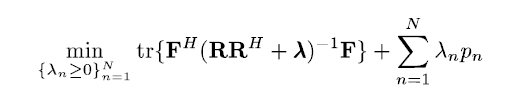# How can I solve this

I want to solve a problem like thisthe variable is a diagonal matrix lambda and here is my code

``````cvx_begin
variable lambda(N,N) diagonal;
minimize(trace(F'/(R*(R')+lambda)*F) + sum(lambda*p));
cvx_end
``````

but I get the error which is as follows

Disciplined convex programming error:
Matrix divisor must be constant.

z = mtimes( x, y, ‘rdivide’ );

minimize(trace(F’/(R*(R’)+lambda)F) + sum(lambdap));

I wonder what went wrong and hope somebody could help me with this.

Well, I guess you should use A*inv(B) instead of A / B.

My solution at Generalizing "trace_inv" for matrix quadratic forms does what you need.

Note: @trump_mink’s solution is not consistent with CVX’s rules.

@Mark_L_Stone’s solution is right. For a matrix containing a cvx variable, cvx can’t inverse matrix by the function inv()